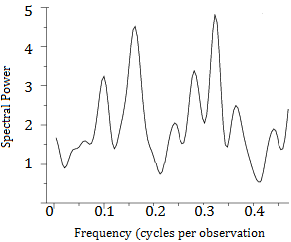# Spectral Plot: Definition

Share on

## What is a Spectral Plot?A spectral plot is a graph which allows us to examine the cyclic structure, in the frequency domain, of a time series. A time series represents a progression of some parameter in time. We can think of it as a function of time, but it is typically random; We can’t predict exactly what it will do in the future; only what it might do.

In technical terms, a spectral plot is a smoothed Fourier transform of the auto-covariance function of the time series. But you don’t need to understand that sentence to understand how to read and use spectral plots.

In a spectral plot:

• The horizontal axis is the frequency—recorded in cycles per observation, which is the same as cycles per unit time when time is defined as the distance between points.
• The vertical axis is smoothed variance. This is computed from the data by a series of rather complicated computations which are typically left to a computer.

## Importance of Spectral Plots

Spectral plots are important because they are the prime technique used in statistics and data analysis for determining the cyclic nature of univariate (one-variable) time series in the frequency domain. In typical frequency domain analysis, a run chart is first generated, followed by a spectral plot.

The spectral plot answers such questions as:

• Whether or not there is a dominant cyclic frequency,
• What that cyclic frequency might be,
• How many cyclic components there are.

Most general statistical software packages have the ability to compute and graph spectral plot; Since the computation is involved they are now rarely figured out by hand.

## References

NIST/SEMATECH e-Handbook of Statistical Methods, 1.3.3.27. Spectral Plot. retrieved from https://www.itl.nist.gov/div898/handbook/eda/section3/spectrum.htm on April 5, 2018
Donman, Kenneth. Spectral Analysis: A Summary of the Theory and Techniques